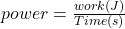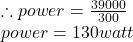## If a football player does 39000 J of work, how much power does the football player exert in 5 minutes

Question

If a football player does 39000 J of work, how much power does the football player exert in 5 minutes

in progress 0
6 months 2021-08-13T22:22:46+00:00 1 Answers 0 views 0

## Answers ( )

Power = 130 watt

Explanation:

Power is described as the ability to do work, it is also defined as the amount of work in Joules done in a given time in seconds. Mathematically, it is represented as:In this example, power is calculated as follows:

Work = 39000 J

Time = 5 minutes

converting the time from minutes to seconds:

1 minute = 60 seconds

∴ 5 minutes = 60 × 5 = 300 secondsN:B the unit for power can also be represented as Joules/seconds or J/s or JS⁻¹